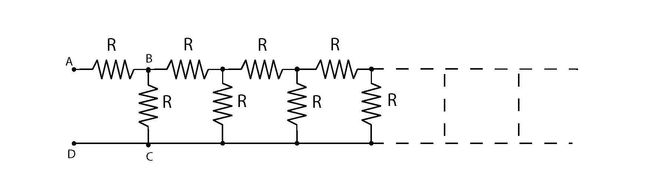In the figure above, the effective resistance between the points $A$ and $D$ of the infinite ladder can be represented as $\frac{a+b\sqrt{c}}{2} R$, where $c$ is square free.
Find the value of $a+b+c+2$.